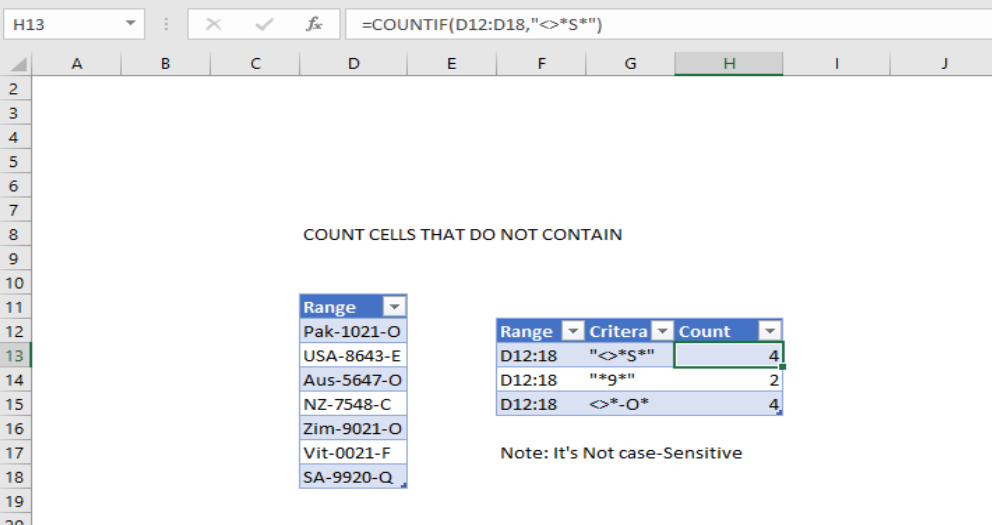Get instant live expert help with Excel or Google Sheets“My Excelchat expert helped me in less than 20 minutes, saving me what would have been 5 hours of work!”

#### Post your problem and you’ll get expert help in seconds.

Your message must be at least 40 characters
Our professional experts are available now. Your privacy is guaranteed.

# How to Count Cells That Do Not Contain Certain Criteria

Want to learn more about how to count cells do not contain using Excel spreadsheet?? This post will give you an overview of how to use COUNTIF formula functions to get the number of cells that do not contain using Excel.

## Count cells that do not contain in Excel

### Syntax(Generic Formula)

`=COUNTIF(range,”<>*txt*”)`

### How COUNTIF & COUNTIFS work

Excel has many syntax for a user who needs to specify on counting the total amount of cells with single or multiple criteria. For example, if you want to count cells based on more than one or more criteria, you can use the COUNTIF or COUNTIFS functions in Excel.Figure 1: Example of count cells that do not contain

To count cells that do not contain, you can use the COUNTIF function. In the example shown, the formula in H13 is

`=COUNTIF(D10:D18”<>”S”)`

### Difference Between COUNTIF & COUNTIFS

The difference between COUNTIF and COUNTIFS function is about the total number of condition that applies. While COUNTIF is designed to count the cells with a single condition, COUNTIFS function will let you have several criteria indifferent or in the same range as you wish.

`=COUNTIFS(range”<>*?*”,rang”?*”)`

By this tutorial, you have learned how to count the number of cells which don’t contain a specific text string.

Still need some help with Excel formatting or have other questions about Excel? Connect with a live Excel expert here for some 1 on 1 help. Your first session is always free.

### Did this post not answer your question? Get a solution from connecting with the expert.Another blog reader asked this question today on Excelchat:
Solution examplesI have a spreadsheet that contains a list of names/IDs and I want to generate a formula that inserts into a cell the number of times a name or ID appears on that list. Either insert a column into that spreadsheet or transfer that info into a column on a different spreadsheet. Either way would be fine.
Solved by O. L. in 18 minsI need a formula to count the number of rows that contain at least one of two possible phrases, but not give me the total number of instances that both phrases occur overall.
Solved by E. B. in 33 minsHello, I have a spreadsheet with 3159 rows. One column consists of 9-digit numbers in each of the 3159 rows. I need the formula that can calculate how many of those numbers appear more than once.
Solved by D. H. in 56 minsi need a formula to find values in column f in worksheet 1 and count them.
Solved by B. A. in 20 minsi have a table with Dates, First names , Last names and i want to count how many times a name occurs, but if a name occurs more than once on a particular date i need to only count it once. this is on excel and not google sheets
Solved by F. H. in 31 mins## Subscribe to Excelchat.coAnother blog reader asked this question today on Excelchat: